A funny series

One of the reasons I posted a funny number was to tell you the story of a funny series I discovered by chance. It had to do with my fascination for simple constructs like $j^j$ giving unexpected results like ${\rm e}^{-\pi/2}$. I always wondered: are there more numbers connected to powers of $j$ or numbers to the power of $j$?

One day I was using Matlab for a tutorial. Before it started I had the Laptop set up and Matlab running and 10 minutes to fill. Waiting for the students to come I played in Matlab: I made a random complex number, raised $j$ to the power of it, then again $j$ to the power of the last result and so on. In other words, I kept typing x = j^x and looked at x. After a little while I got (rounded to 4 digits) $0.4383 + j 0.3606$ which seemed to be a stationary point since $j^{(0.4383 + j 0.3606)} = 0.4383 + j 0.3606$. I started with another random number and got the same result. This made me think: is this stationary point unique? Do we reach it no matter where we start? If so, does it have an analytical expression? Is there anything else special about it?

I was ripped out of my train of thoughts when the seminar started. I forgot about my little experiment for quite some time until quite recently I found the old script by chance. I really wanted to find out more about that special number.

What is it I am really trying to find? It’s the limit of a recursively defined sequence: it is $\lim_{n\rightarrow \infty} x_{n}$ where

$$x_{n} = j^{x_{n-1}}$$

and $j^x$ refers to the principal value, i.e., $j^x = {\rm e}^{j \cdot \pi/2 \cdot x}$. From this it seems that the sequence can easily be generalized to

$$x_{n} = {\rm e}^{\alpha \cdot x_{n-1}},$$

where $\alpha \in \mathbb{C}$ and the previous series corresponds to the special case $\alpha = j \cdot \pi/2$. This has got to be something veeery fundamental I thought and it does generate the “magic” stationary point I had found earlier.

Unfortunately I didn’t get much further on the analytical side. You can certainly expand $x$ into $y+jz$ so that for $\alpha = j \cdot a$ (purely imaginary), ${\rm e}^{\alpha \cdot x}$ becomes

$${\rm e}^{- a \cdot z + j \cdot a \cdot y} = {\rm e}^{-a \cdot z} \cdot \cos(a \cdot y) + j{\rm e}^{-a \cdot z} \cdot \sin(a \cdot y)$$

but I do not see this going anywhere. A quick google search turned up a lot of results on linear recurrence relations but the one I had is clearly non-linear.

As of now I have no analytical expression for the stationary point for any value of $\alpha$ (trivial solutions like $\alpha=0$ let aside).

So I played a bit more with it in Matlab. It seems that different values of $\alpha$ also give rise to stationary points. For instance, $\alpha=j$ gives $0.5764 + j \cdot 0.3743$. Here is a plot with empirically found fixed points for $\alpha = j \cdot a$, varying $a$ from $0$ to $1.96$ (the series starts to form cycles for $a$ larger than $\approx 1.96125$):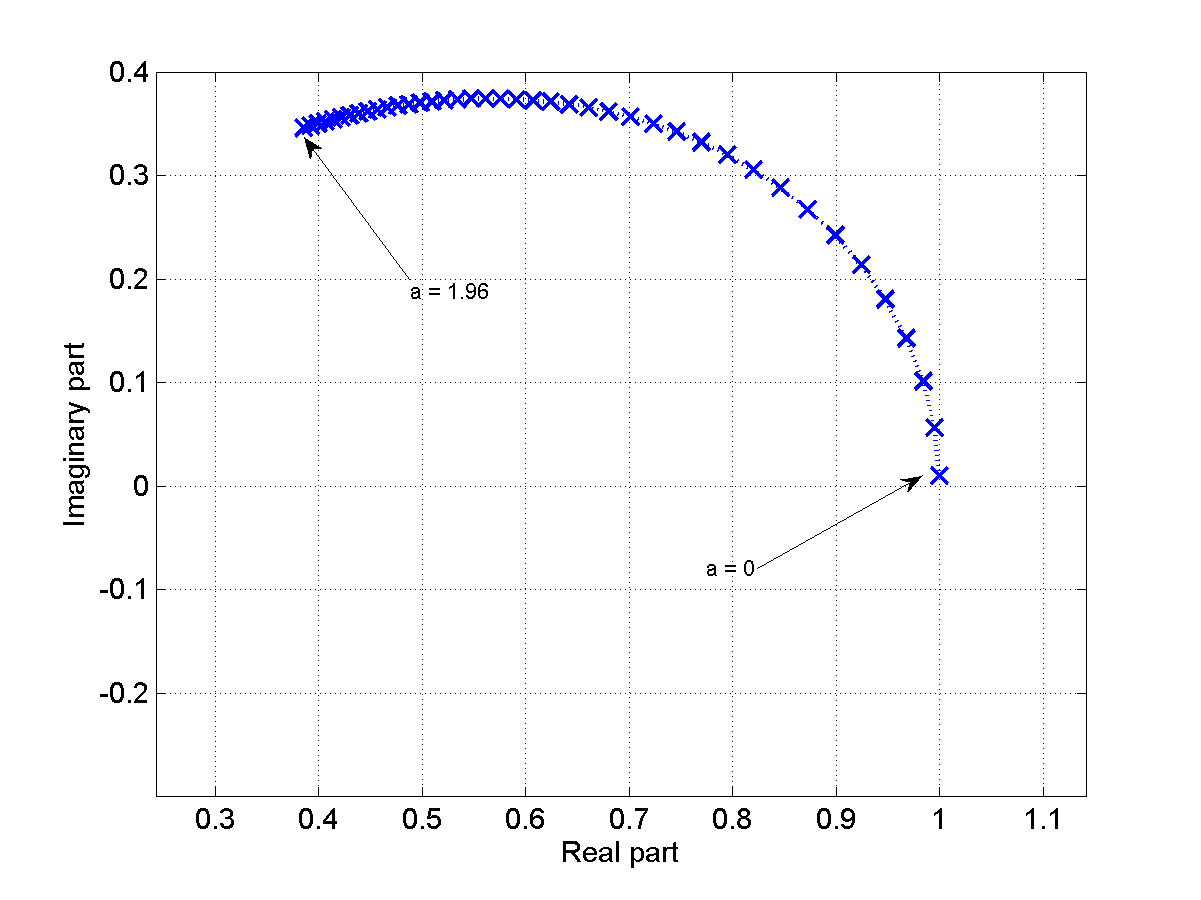It does not seem to be a circle. This is purely empirical, I have no clue if/how one could find these values analytically (but this would be very nice).

While playing with these numbers I realized that my original observation that one would always converge to one fixed point regardless of the initialization was actually wrong. For some points, the series diverged to infinity. This sparked the question where these points would be located on the complex plane. So I made a second set of experiments: I started the series from initial points $x_0$ chosen in a regular grid in the complex plane, let the series run for a predefined maximum number of iterations and observed what it does: (a) does it diverge to infinity or (b) does it converge to the fixed points I found earlier (i.e., get within a predefined threshold $\delta$ of it)?

I began with $\alpha = j\cdot \pi/2$ (corresponding to the $x_{n+1} = j^x_{n}$ it all started with) and a cartesian grid in ${\rm Re}(x_0)$ and ${\rm Im}(x_0)$ for the initial points. And then, I got this: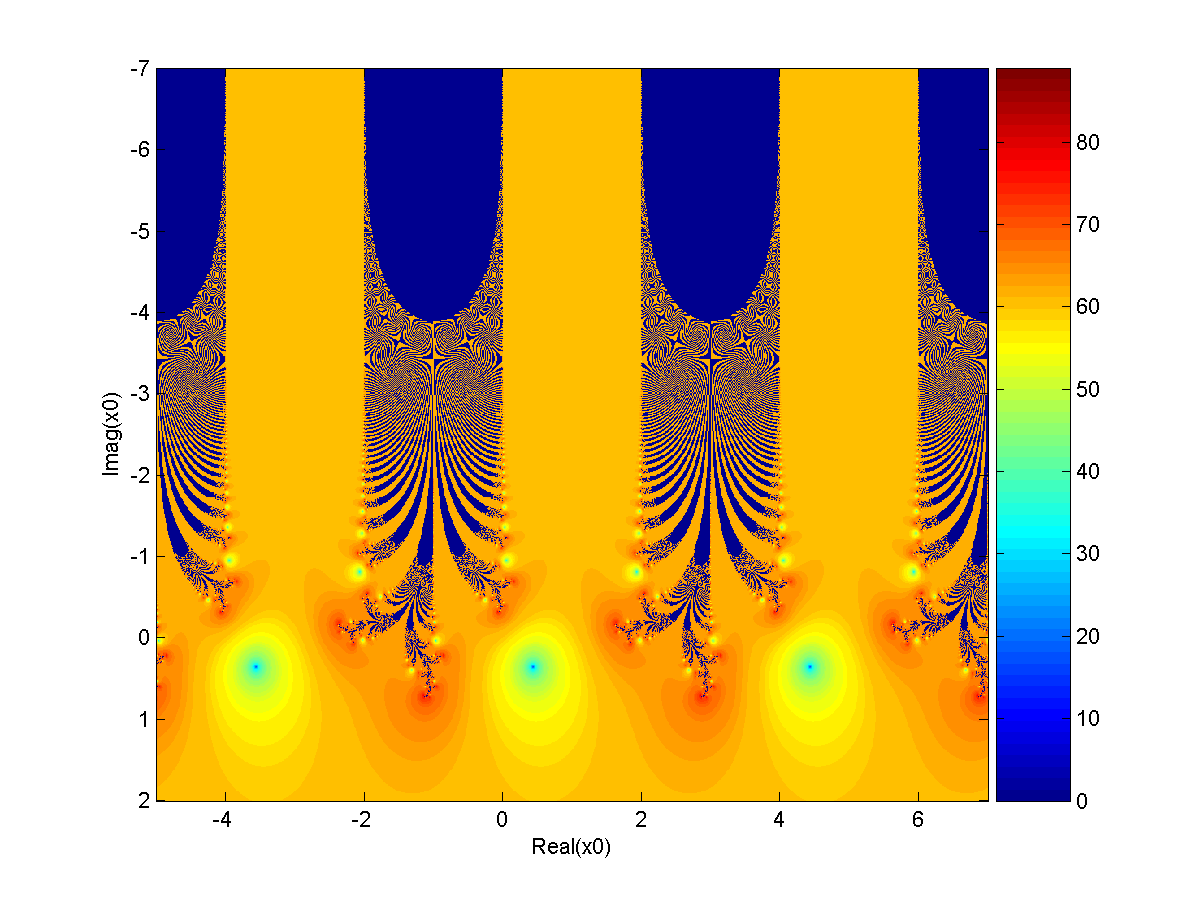The two axis refer to the real part and the imaginary part of our initial point $x_0$. The color indicated the number of iterations until the fixed point is reached up to an accuracy of $10^{-3}$. We notice the periodicity in ${\rm Re}(x_0)$: this is not surprising since the first step in the series gives

$x_1 = {\rm e}^{j \cdot \pi/2 \cdot x_0} = {\rm e}^{-\pi/2 \cdot {\rm Im}(x_0)} \cdot {\rm e}^{j \cdot \pi/2 \cdot {\rm Re}(x_0)}$

and therefore, adding an integer multiple of 4 to ${\rm Re}(x_0)$ gives the same $x_1$. I found the shape looks very interesting and started zooming in.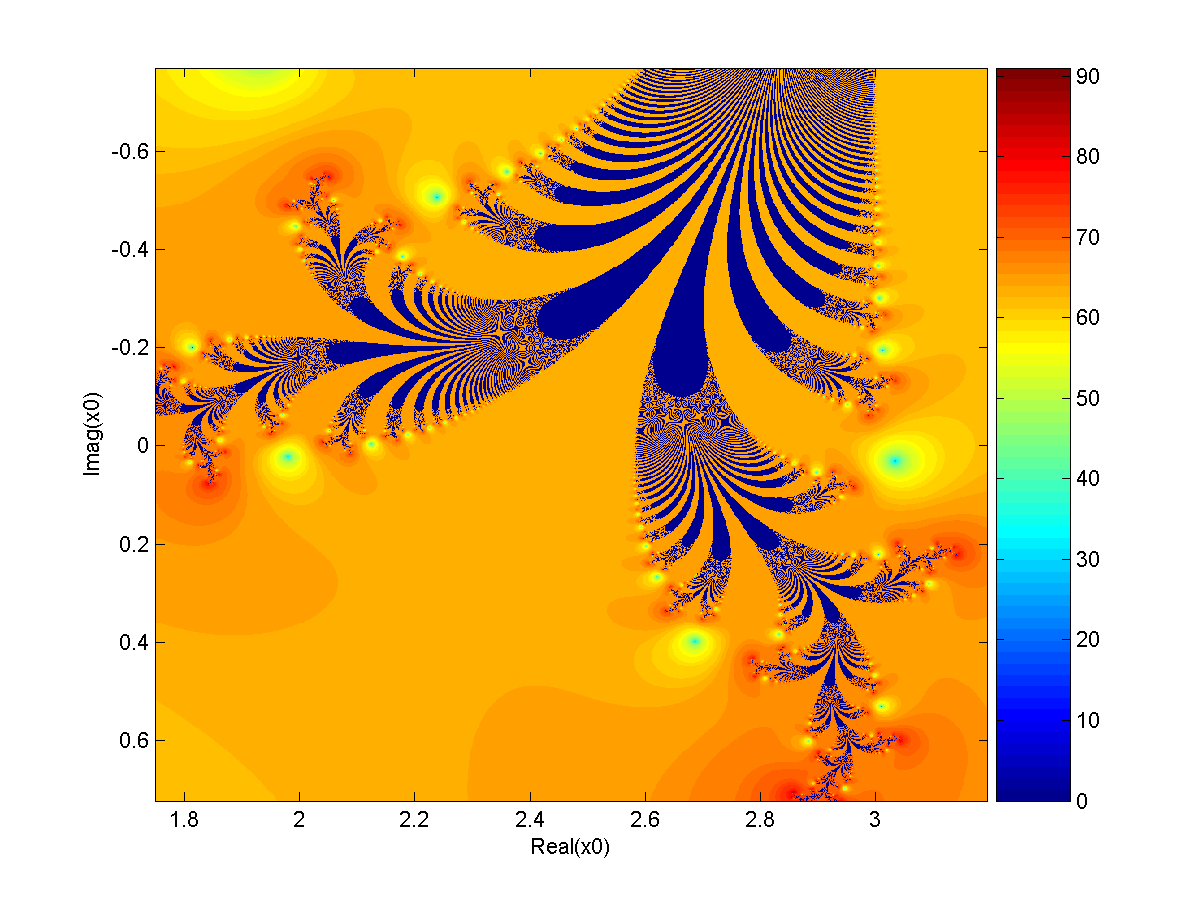Even further…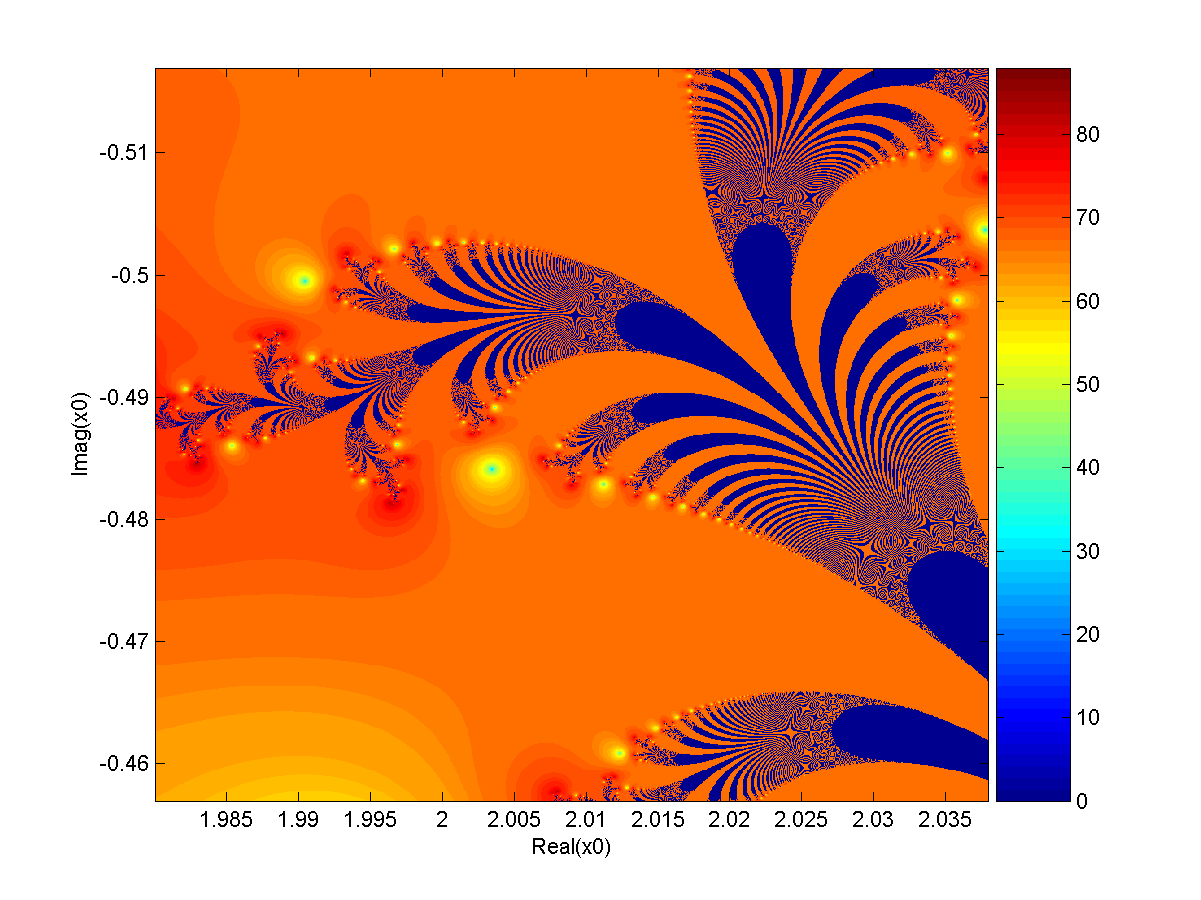This is already a 200x zoom on both axis compared to the original one. Yet more and more details are revealed that look similar to the original ones – we seem to have self-similarity here. I’m not sure if that’s enough to call this a fractal but I’d like to claim so until someone convinces me otherwise.

I have since played with various values of $\alpha$, kept zooming in and looking at the result. So far it seems that the level of details keeps increasing as $\alpha$ approaches $2 \cdot j$. After about $1.961 \cdot j$ it becomes hard to still draw a picture because instead of unique attractors the fractal seems to run into limit cycles consisting of a discrete number of points. There still seems to be a fractal-looking region of divergence but I’m mostly guessing here.

Here is one  for $\alpha = j \cdot 1.96$: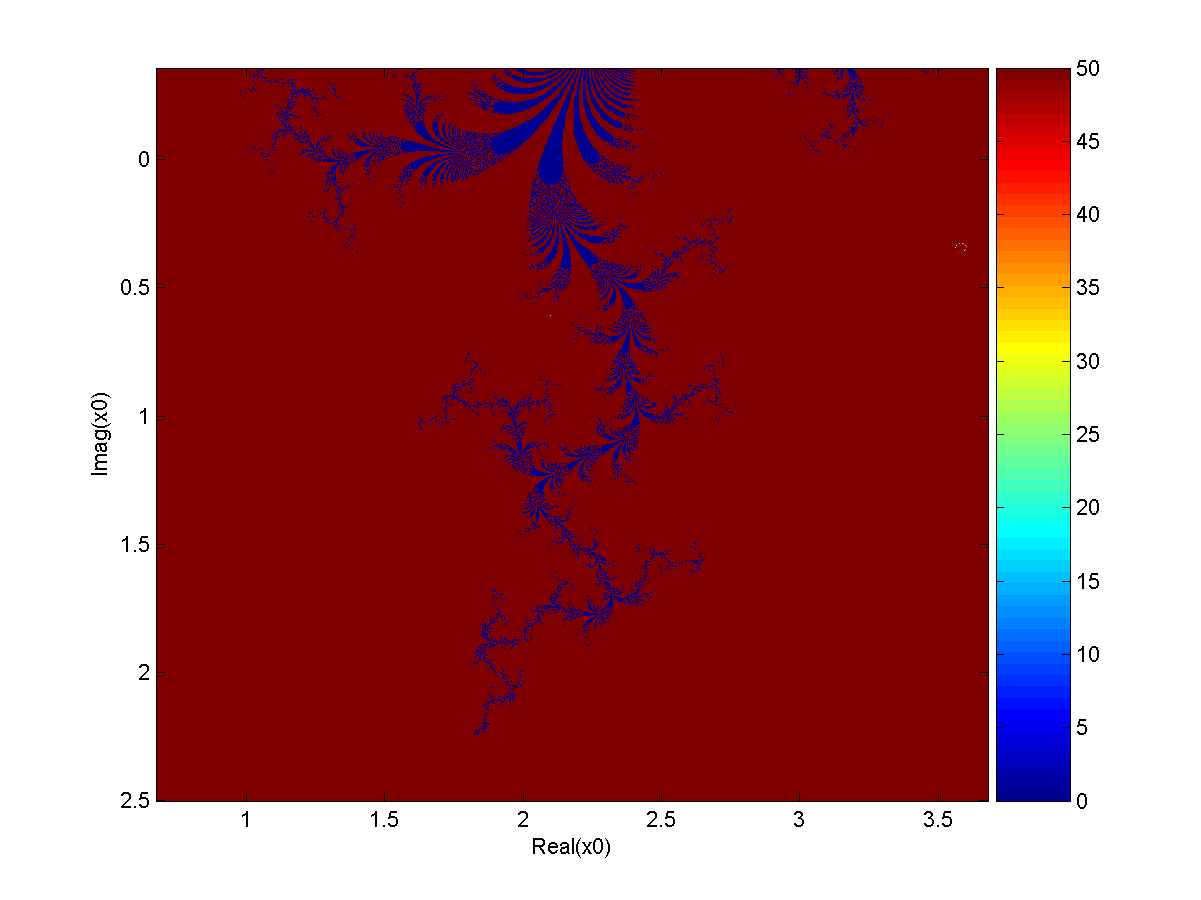I chose 50 as the maximum number of iterations here, the picture does not change much if I increase it to 1000 or so (it just takes much longer to render).

Here is my best attempt for $\alpha = j \cdot 1.963495$:I display the plane of initial points in polar instead of cartesian coordinates (for cosmetic reasons only) so this one is periodic in the vertical direction and truncated on the right side horizontally. The maximum number of iterations was set to 2000 here, increasing it further does have an impact on the larger red areas that get filled more and more. I tried to count how long it takes until a limiting cycle is reached but this is based on very rough heuristics and might be wrong. I still enjoy the look of the result.

It’s really a lot of fun to “explore” this little world, especially if you’ve never seen it before and you’re not sure if anyone else ever has. It’s quite possible these fractals are actually well-known, I just don’t know what I should search for. In light of this it would be really great if anyone could give me any hint that brings me closer to any of these open questions:

• Is this class of series known, does it have a name, is there any published material on it?
• How can we compute the fixed points of the series for a given value of $\alpha$?
• Are the resulting images that divide converging from diverging initial points actually a fractal? Does it possibly also have a name, has it been studied before?
• What happens beyond the “magic” breaking point where the series starts running into limit cycles comprising of a discrete number of points? Does the breaking point have any special meaning? Can the limit cycles be described analytically?

If you have any idea to any of these please leave me a comment!